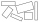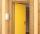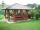# Rectangle + comparing - math problems

1. Two 2D shapesDecide which shapes have more area:   (a) a square of 8cm side; or   (b) two rectangles with sides 5cm and 15cm? Write result as 1 or 2 (rectangles)
2. The perimeter of the rectangleThe length, l, of a rectangle is 4 inches greater than its width, w. The perimeter of the rectangle is at least 30 inches. What inequality shows the range of possible widths of the rectangle?
3. Paper boxCalculate whether 11 dm² of paper is sufficient for gluing a box without a lid with bottom dimensions of 2 dm and 15 cm and 12 cm high. Write result as: 0 = No, 1 = Yes
4. The doorThe door to the apartment has a height 2 m and a width 80 cm. Calculate how many cm of sealing tape is needed to seal them. Will there be enough packaging in which there are 5 m tape (write 0 = no, 1 = yes)?
5. PavilionThe rectangular pavilion with dimensions 3.5 m and 2.75 m to be paved with square tiles of side 25 cm price of CZK 22 per 1 piece or rectangular tiles with sides of 20 cm and 15 cm in the price of CZK 11 per 1 pc. Which solution is cheaper (write its pric
6. Built-up areaJohn build up area 5 x 7 = 35 m2 with building with a wall thickness 30 cm. How many centimeters would have to subtract from thickness of the walls that built-up area fell by 9%?
7. CylindersArea of the side of two cylinders is same rectangle of 33 mm × 18 mm. Which cylinder has a larger volume and by how much?

We apologize, but in this category are not a lot of examples.
Do you have an interesting mathematical word problem that you can't solve it? Submit math problem, and we can try to solve it.

We will send a solution to your e-mail address. Solved examples are also published here. Please enter the e-mail correctly and check whether you don't have a full mailbox.

Please do not submit problems from current active competitions such as Mathematical Olympiad, correspondence seminars etc...